Next: 1.4 Plan of the Up: 1 Introduction Previous: 1.2 Generators, relations and   Contents

Subsections

## 1.3 Parenthesized tangles

Summary. As an example where the scheme of Section 1.2 has been successfully used, and also in order to display some formulas for later use in this article, we give a very quick reminder of parenthesized tangles and the pentagon and hexagon relations and their syzygies, along the lines of [BN3,BN5,LM].

The papers [BN3,BN5,LM] introduce an algebraic context within which the scheme of Section 1.2 is used to construct a universal finite type invariants of links. The algebraic context'' there is the structure of a category with certain additional operations. Rather than defining everything in full, we will just recall some key notions, pictures and formulas here.The category PaT of parenthesized tangles'', (the algebraic structure which we wish to represent, likein Section 1.2) is the category whose objects are parenthesizations such as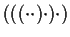or, and whose morphisms are tangles with parenthesized top and bottom. See the picture on the right, which also illustrates how parenthesized tangles are composed.The category PaT carries some additional operations. The most interesting are the strand addition on the left/right'' operations, and the strand doubling operations (illustrated on the right). More details are in [BN3,BN5,LM].

Likewise, one can set up a category Pa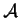of parenthesized chord diagrams'', that captures the symbols'' of singular'' parenthesized tangles as in Equation (1). The category Pasupports the same additional operations as PaT, and one may wish to look for structure preserving functors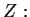PaTPawhich are essential'' in a sense similar to that of Equation (2). In [BN3], this is done following the same generators-relations-syzygies sequence as in Section 1.2:

### 1.3.1 Generators

The category PaT is generated by the morphisms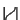and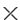. We set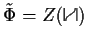andand then reconsider these morphisms in Paas elementsand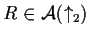, where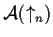denotes the usual space of chord diagrams modulo,and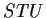relations on a skeleton made ofvertical lines.

### 1.3.2 Relations

There are several relations beweenandas elements of the algebraic structure Pa, as listed in [BN3]. The most prominent of those are the pentagon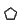and the two hexagon relations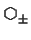, displayed in Figures 2 and 3.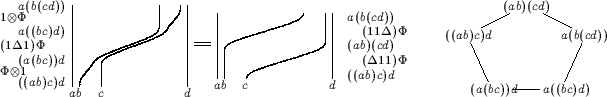Now let us assume that we already foundand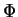so that the relations between them corresponding toandare satisfied up to degree 16 (say), and letandbe the degree 17 errors in these equations (compare with Equation (4)). That is, modulo degrees 18 and up we have (notaion as in [BN3], compare with [BN3, Equations (10) and (11)]):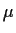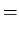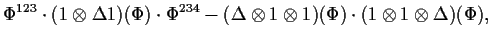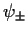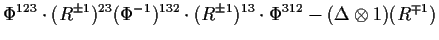Proceeding as in Equation (5) we setandwithand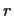of degree 17, and like in Equation (6) we get (compare with [BN3, Equations (12) and (13)]):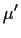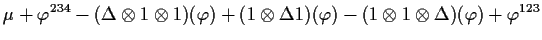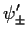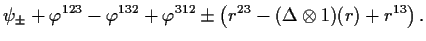Thus we are interested in knowing whether the tripleis in the image of the linear map### 1.3.3 Syzygies

On like in Section 1.2, the trick is to use syzygies between theandrelations to reduce the problem to the computation of a homology groupwhereis some other linear map, for which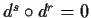and. Again, this was carried out in full in [BN3]. Here we only reproduce the four syzygies we need to use (see Figure 4) and the resulting map(the four components ofcorrespond to the syzygies in Figure 4 in the order; the symbol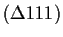denotesetc.; compare with [BN3, Equations (15-19)]):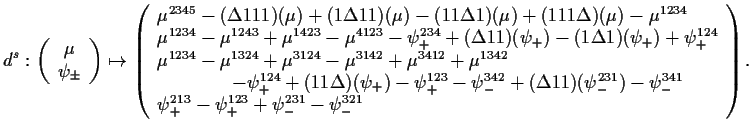Next: 1.4 Plan of the Up: 1 Introduction Previous: 1.2 Generators, relations and   Contents
Dror Bar-Natan 2001-07-23